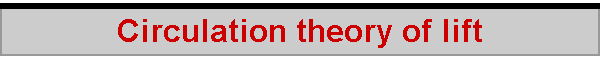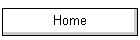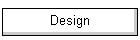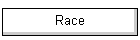The aerodynamicist's way of understanding the lift produced by a lifting surface is called the "circulation theory of lift".  This page considers a "simple" lifting surface of infinite length.  A wing of finite length is considered in the Lifting line theory of lift, which is the development of the circulation theory to deal with more realistic situations.  The circulation theory is similar to the momentum theory of lift, in that it too considers the reaction force on the wing (sail, fin) as a result of the wing "pushing" the air (water) downwards (sideways), but it constructs a different model of what is happening when air is displaced by the aerofoil.  This is how it works. First, we need to see what is meant by "circulation".  The idea is that, if a fluid is "circulating" around some object, the speed of some particle in the fluid is proportional to the distance to the centre of the circulation.  For a given amount of "circulation", the further away the circulating particles, the slower they move.  The amount of circulation is said to be the speed of the particles times the length of their circulation path.  Suppose their path is a circle.  The amount of circulation, called gamma, is given as 2 pi r, the circumference of the circle, times w, the speed of the circulating particles at some distance r from the centre.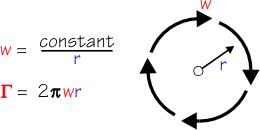The idea is quite an interesting one.  Let us imagine we have gamma = 12.  This means that, at a distance of 1 from the centre, the particles are circulating with a speed of 12.  At a distance of 2, they circulate with a speed of 6.  At a distance 3, with a speed of 4, at a distance 4, with speed 3, and so on.  All of this is given when we say, the amount of circulation is 12. Here is a diagram of a foil moving through a fluid at a speed of V.  If we look at a rectangular path around this foil, "h" units high and "k" units long, and ask about the amount of circulation we see, we can calculate it by multiplying the speed of the particles at each point around the path and adding this up. Starting at the top of the rectangle, we see that the particles are travelling at a speed V, and they do so over the distance k;  the circulation here is "Vk". Down the right-hand side, the particles now show an average downwash speed w, along a distance h;  circulation here is "wh". Along the bottom, the speed of the particles is -V (relative to the direction of the path we are following), and they travel at this speed for the distance k, so circulation here is "-Vk".  Finally, up the left-hand side, we see that none of the particles are moving up or down, so their speed vertically is zero, 0.  The length of the path over which they travel at, er, zero speed is h, and so the circulation here is "0".  Add up these regions of circulation, and we find that gamma, the overall amount of circulation is simply "wh".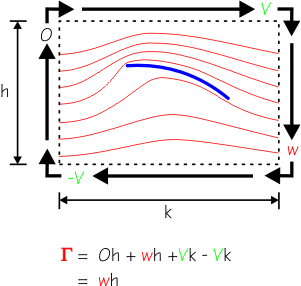The amount of circulation around the foil is given by the amount of downwash times the distance over which the downwash occurs. This is one of the reasons why the circulation theory is conceptually attractive, since it gives us a model of how the downwash is strongest at the aft edge of the aerofoil and then weakens and dissipates the further away we measure it.  The momentum theory of lift is unable to give a coherent account of this feature of downwash. We used the rectangle as a simple and intuitive way to derive the amount of circulation around a path of interest.  The path of interest is, more realistically, conceptualised as a circle, and we associate the circulation wh to be the circulation around a circle whose circumference is h.  If the circle's diameter is c, its circumference h is given by the usual formula, h = pi * c. Let's now look at a wing (sail, fin) moving through the air (water) with velocity V.  Because of the wing's action, it causes some "circulation" in the air as the air passes by.  This is the same as saying that it causes some downwash.  We consider a portion of the wing, with a span of b and a chord of length c, causing some downwash with velocity w that acts around a path of length h.  The circulation is gamma, equal to w times h, and our task is to deduce the lift of the wing.The lift force of the wing is given by considering that it has deflected the air downwards, in a unit of time, giving it a downwash speed w.  This lift force is the mass of the air which has been deflected times the downwash speed.  The mass of air deflected in unit time is its density, rho, times its volume, which is given by V * b * h.   At this point in the derivation of the theory, we are using a version of the momentum theory of lift.  The momentum theory conceptualised the volume of air as a cylinder of length V with a circular cross-section of diameter b.  Here we consider a physically more plausible volume of air as a cube of length V with width b and height h. We know that the amount of circulation around the wing, gamma, is equal to the downwash speed w, times the length of the path over which it operates, h.  This gives us the fundamental result of the circulation theory -- lift, the force acting at right angles to the air stream, equals the density of the air times the velocity of the air times the circulation, for a wing of unit span.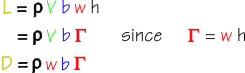A similar result holds when we consider the drag, which is the amount of force acting at right-angles to the average downwash.  Drag equals the density of the air times the downwash of the air times the circulation, for a wing of unit span.  Notice we say "wing of unit span".  This is because we consider the wing here to go on forever -- an infinite wing.  The circulation around a real wing, of a fixed span, is considered in the lifting line theory of lift. According to the circulation theory, we can now derive the lift coefficient from knowledge of the downwash.  Let us consider the circulation around the wing, from leading edge to trailing edge, and consider the circulation path as a circle whose diameter is the chord, c, of the wing.  We calculate the lift using the lift coefficient in the usual way, and set that equal to the lift calculated using the theory of circulation.  (For convenience, we calculate the circle circumference as 2*pi times the radius, where the radius is c/2.  That is, we set h = 2*pi*c/2.) We then obtain one of the fundamental results of the circulation theory of lift, that the lift coefficient is proportionate to the ratio of the downwash speed to the air speed.  The higher the downwash relative to the speed of the air, the greater the lift coefficient.If we consider the case of a simple flat plate, and imagine that the plate deflects the air downwards, we can estimate that the angle of deflection is the angle of attack of the flat plate, alpha.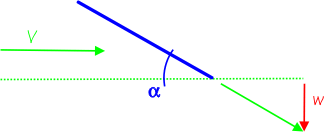And, we can estimate that, for small alpha, the angle of deflection is approximately w divided by V .  In this case, the lift coefficient for a flat plate is 2 pi times the angle of attack.  When we convert from radians to degrees, this says that the lift coefficient increases by 0.11 for every degree of attack.  In fact, this result holds (in theory!) for more than a flat plate -- it holds for all lifting surfaces.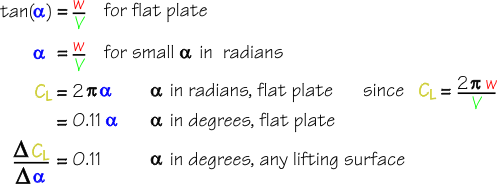A second fundamental result of the circulation theory of lift is found by expressing the lift coefficient as a function of the circulation produced around the wing.This tells us that, for a given circulation gamma, the lift coefficient CL increases as the wing chord c decreases.  Because we have developed the theory so far by considering a part of an "infinite" unit wing of constant chord c all along its span, this result is not yet of much interest.  But a real wing typically has a taper, such that its chord decreases from root to tip.  Other things being equal, this circulation theory result explains that CL increases along the taper, probably rather undesirably, and Lifting line theory is needed to give a clear account of what happens at the wing tip and why an elliptical wing planform is so important.  By using the circulation theory of lift, lifting line theory gives an account of the wingtip vortex that is otherwise outside the scope of the momentum theory of lift.  This ability to account for the wingtip vortex (and, in a sail, the sail foot vortex) is given by the circulation theory's treatment of downwash using vortex mathematics.  In a fundamental respect, however, both circulation theory and lifting line theory are based on momentum theory.  All three theories consider a volume of fluid being deflected by the aerofoil to give downwash.  In their derivation, they use the ratio of downwash to velocity to approximate the angle of attack for small angles and estimate the lift coefficient. 2022-07-13